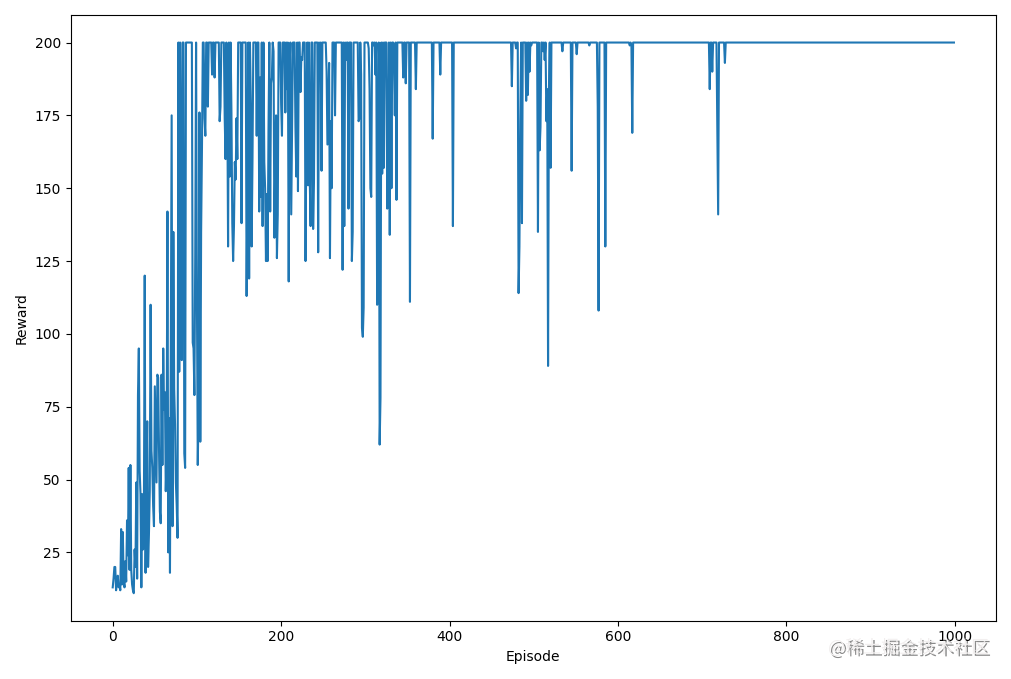# PyTorch強化學習——策略梯度演算法

## 2. 使用策略梯度演算法解決CartPole問題

n_state = env.observation_space.shape print(n_state)

n_action = env.action_space.n print(n_action)  定義run_episode函式，在此函式中，根據給定輸入權重的情況下模擬一回合CartPole遊戲，並返回獎勵和計算出的梯度。在每個時間步中執行以下操作： - 根據當前狀態和輸入權重計算兩個動作的概率probs- 根據結果概率取樣一個動作action- 以概率作為輸入計算softmax函式的導數d_softmax，由於只需要計算與選定動作相關的導數，因此：

$$\frac {\partial p_i} {\partial z_j} = p_i(1-p_j), i=j$$

• 將所得的導數 d_softmax 除以概率 probs，以得與策略相關的對數導數d_log
• 根據鏈式法則計算權重的梯度 grad

$$\frac {dy}{dx}=\frac{dy}{du}\cdot\frac{du}{dx}$$

• 記錄得到的梯度 grad
• 執行動作，累積獎勵並更新狀態 python def run_episode(env, weight): state = env.reset() grads = [] total_reward = 0 is_done = False while not is_done: state = torch.from_numpy(state).float() # 根據當前狀態和輸入權重計算兩個動作的概率 probs z = torch.matmul(state, weight) probs = torch.nn.Softmax(dim=0)(z) # 根據結果概率取樣一個動作 action action = int(torch.bernoulli(probs).item()) # 以概率作為輸入計算 softmax 函式的導數 d_softmax d_softmax = torch.diag(probs) - probs.view(-1, 1) * probs # 計算與策略相關的對數導數d_log d_log = d_softmax[action] / probs[action] # 計算權重的梯度grad grad = state.view(-1, 1) * d_log grads.append(grad) state, reward, is_done, _ = env.step(action) total_reward += reward if is_done: break return total_reward, grads

for e in range(n_episode): total_reward, gradients = run_episode(env, weight) print('Episode {}: {}'.format(e + 1, total_reward)) for i, gradient in enumerate(gradients): weight += learning_rate * gradient * (total_reward - i) total_rewards.append(total_reward) 然後，我們計算通過策略梯度演算法獲得的平均總獎勵：python print('Average total reward over {} episode: {}'.format(n_episode, sum(total_rewards)/n_episode)) 我們可以繪製每個回合的總獎勵變化情況，如下所示：python plt.plot(total_rewards) plt.xlabel('Episode') plt.ylabel('Reward') plt.show()print('Average total reward over {} episode: {}'.format(n_episode_eval, sum(total_rewards_eval)/n_episode_eval))

# Average total reward over 1000 episode: 200



## 相關連結

PyTorch強化學習——PyTorch+Gym強化學習環境搭建

PyTorch強化學習——隨機搜尋策略

PyTorch強化學習——爬山演算法